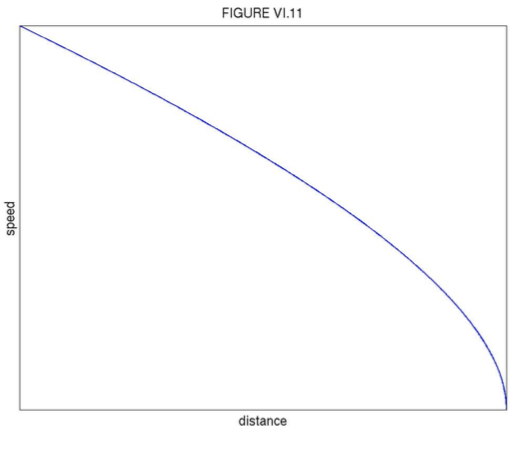Skip to main content
$$\require{cancel}$$

# 6.3C: Body thrown vertically upwards, initial speed $$v_{0}$$

•• Contributed by Jeremy Tatum
• Emeritus Professor (Physics & Astronomy) at University of Victoria

If we measure $$y$$ upwards from the ground, the equation of motion is

$\ddot{y}= - g - \gamma v = -\gamma (\hat{v}+v). \tag{6.3.24}\label{eq:6.3.24}$

The first time integral is

$v = -\hat{v} + (v_{0}+\hat{v})e^{- \gamma t} \tag{6.3.25}\label{eq:6.3.25}$

and this is shown in Figure VI.9.It reaches a maximum height after time $$T$$, when $$v=0$$(at which time the acceleration is just $$-g$$):

$t + \frac{1}{\gamma}\ln(1 + \frac{v_{0}}{\hat{v}}). \tag{6.3.26}\label{eq:6.3.26}$

The second time integral (obtained by writing $$v$$ as $$\frac{dy}{dt}$$ in Equation $$\ref{eq:6.3.25}$$) and the space integral (obtained by writing $$\ddot{y}$$ as $$v\frac{dv}{dy}$$ in the equation of motion) require some patience, but the results are

$y = \frac{(v_{0}+\hat{v}}{\gamma}(1-e^{-\gamma t}- \hat{v}t, \tag{6.3.27}\label{eq:6.3.27}$

$v = v_{0} - \gamma y -\hat{v}\ln(\frac{\hat{v}+v_{0}}{\hat{v}+v}). \tag{6.3.28}\label{eq:6.3.28}$

These are illustrated in Figures VI.10 and VI.11.